## Wednesday, 22 December 2010

### Extending the Binomial Distribution

Almost every high school student is exposed to the binomial distribution in some form. They may see it in expanding binomials such as (x+y)4, and they may also come across it as a method of solving simple probability problems... "what is the probability that a family with four children will have three boys and one girl?"

The ability to naturally extend the binomial (or to recognize that the two questions above are interrelated) is probably hampered by the notation used in the "choose" command, or the various combination notations. The normal way for students to address the problems about combinations is to think of one group embedded in the total field. They may useto find three boys, or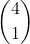to find one girl. Both methods lead to the same calculation,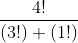but they seem to direct the focus of the learner away from the idea of "three of these and one of those" which would embed the problem firmly in the multinomial distribution. I suspect that if the "choose" or "combination" notation was not used, many students would almost naturally extend the binomial probability problem to similar problems with three (or more) item choices.

For students who have never seen the multinomial I will provide a brief introduction, and a few good links.

Suppose instead of two choices to pick from, a population had three choices..(the extension to four should jump out at you). A spinner has the numbers one, two, and three on it with probabilities of 1/6, 1/3, and 1/2 respectively. What is the probability that in ten spins you would get 2 ones, 3 twos, and five threes. The probability is simply given by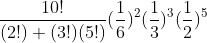The association between the number of things selected in all (10) and the number and probability of the individual partition seems to be naturally extendable to any number of items. Keep in mind that, like the binomial, this requires that the probability on each draw is unchanged... we are drawing with replacement or from an "infinite" pool. This does require that the sum of all the probabities add up to one

You can use this to extend the expansion of a binomial to the expansion of any polynomial to a power. To make this clear to new learners, I will go back to the idea that (x+y)4 is related the probability of three boys and one girl in a family of four children. To do that, I want to give a verbal expansion of (x+y)4, but instead of x and y I will use b and g for (boys probability of birth and girls probability of birth... well, they might not be 1/2). The expansion of (b+g)4 will give the probability of every possible outcome, 4 boys and no girls, 3 boys and one girl, two boys and two girls, one boy and three girls, and no boys and four girls. Each term in the expansion represents one of these cases. For four boys and no girls, we have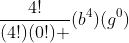, this is added to each succeeding term until we end with no boys and four girls. This gives exactly the expansion you would have for (x+y)4 except for the use of b and g as variables.

To extend this to a trinomial we get a few more terms, but we can just attack them systematically as there is no such natural approach as there is in the binomial case. For instance, if we had (a+b+c)2 we could have two a's, two b's, two c's, or ab, ac, or bc so there must be six terms. It may help to think of it as (a+b+c)(a+b+c) and you pick one term from the first trinomial and one from the second to multiply. The three squared terms will have coefficients of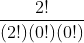which is a big one. The ones where we pick two different ones will be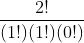, which can occur two ways. So we get a2+b2+c2+2ab+2ac+2bc.

If you want to try (a+b+c)3 then you will get ten terms. In fact for any power n, a trinomial will have the n+1st triangular number which coincidentally is (n+1) choose 2. There is even an extension of Pascal's triangle, called Pascal's tetrahedron that can be used but you have to create it (or at least I do) level by level. I find it usually easier to just do the multinomial coefficients. You can find a pretty good explanation of the tetrahedron here. There is also a good wikipedia page about the multinomial distribution.Anonymous said...

I love this stuff (and teach most of it in my "combinatorics" elective).

Look at this: (a+b+c)^3 implies 3x3 multiplication, so 3 x 3 x 3 = 27 terms before combining like terms:

aaa / aab / aac
aba / abb / abc
aca / acb / acc
baa / bab / bac
bba / bbb / bbc
bca / bcb / bcc
caa / cab / cac
cba / cbb / cbc
cca / ccb / ccc

But I like this piece better:
(a + b)^2 =
aa + ab + ba + bb
(what's the coefficient for ab? <==> how many ways can we rearrange ab)

(a + b)^4 =
aaaa + aaab + aaba + aabb +
abaa + abab + abba + abbb +
baaa + baab + baba + babb +
bbaa + bbab + bbba + bbbb

Coefficient for (a^2)(b^2)?
Same as the number of ways to rearrange aabb.

This is fun.

Jonathan

Pat's Blog said...

A comment on JD2718's nice addition to my post... Not only does " (a+b+c)^3 implies 3x3 multiplication, so 3 x 3 x 3 = 27 terms before combining like terms" because there are ten different terms after the like terms are combined, the sum of the coefficients of those ten terms must add up to .... 27. Now the student should figure what the sum of the exponents of (a+b+c)^4 would be, and how many terms there are,,,